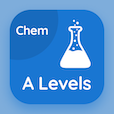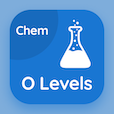A Level Online Courses

A Level Chemistry Quizzes

A Level Chemistry Practice Test 263

The e-Book Mole Calculations Multiple Choice Questions and Answers (MCQs), mole calculations quiz answers PDF chapter 19-263 to study online courses, a level chemistry tests. Study Moles and Equations MCQ trivia questions, mole calculations Multiple Choice Questions (MCQ Quiz) for online college degrees. The e-Book "Mole Calculations MCQs" App Download: mole calculations, intermolecular forces in chemistry, environmental problems caused by nitrogen compounds and nitrate fertilizers, electrode potentials, order of reaction test prep for online colleges that offer certificate programs.

The MCQ "The molecular formula helps in" PDF, Mole Calculations App Android & iOS (Free) with calculating molar mass only, writing balanced equation only, calculating moles, and writing balanced equation and calculating molar mass choices for free online college classes. Practice moles and equations questions and answers, Google eBook to download free sample for colleges that offer certificate programs.

## Chemistry: Mole Calculations MCQ Quiz

MCQ: The molecular formula helps in

A) writing balanced equation only
B) calculating molar mass only
C) calculating moles
D) writing balanced equation and calculating molar mass

MCQ: The strength of an atom to attract electrons increases with the increase in

A) polarity
B) molarities
C) electronegativity
D) none of above

MCQ: Tiny droplets of water (sulfuric acid solution) in clouds are

A) an essential component of Nitrogen cycle
B) not related to nitrogen cycle
C) most damaging in trees of high altitudes
D) none of above

MCQ: Two chemical-related species will undergo Redox reaction if they are in different

A) oxidation states
B) reducing states
C) returning states
D) reversing states

MCQ: Order of reaction can be identified by plotting graphs of

A) reaction only
B) concentration only
C) pH value
D) reaction and concentration

### Class Quizzes: A Level Chemistry Prep Tests

Download A level Chemistry Quiz App, SAT Chemistry MCQs App and O Level Chemistry MCQ App for Android & iOS devices. These Apps include complete analytics of real time attempts with interactive assessments. Download Play Store & App Store Apps & Enjoy 100% functionality with subscriptions!